Open In App
Related Articles
• NCERT Solutions for Class 10 Maths

# Class 10 NCERT Solutions- Chapter 13 Surface Areas And Volumes – Exercise 13.4

### Question 1. A drinking glass is in the shape of a frustum of a cone of height 14 cm. The diameters of its two circular ends are 4 cm and 2 cm. Find the capacity of the glass.

Solution:

Given values:

Height of frustum (h) = 14 cm

Radius of larger circle end (R) == 2 cm

Radius of smaller circle end (r)== 1 cm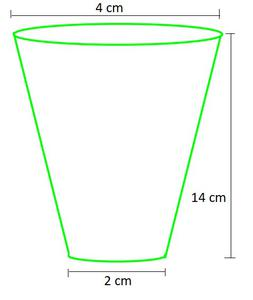Capacity of frustum-shaped glass = Volume of Frustumπh (r2 + R2 + rR)× π × 14 ((1 × 1) + (2 × 2) × (2 × 1))× 14 × 7                     (taking π=)= 102.67 cm3

Hence, the capacity of frustum-shaped glass = 102.67 cm3

### Question 2. The slant height of a frustum of a cone is 4 cm and the perimeters (circumference) of its circular ends are 18 cm and 6 cm. Find the curved surface area of the frustum.

Solution:

Slant height of frustum (l) = 4 cm

Let radius of smaller circle end = r

Let radius of larger circle end = R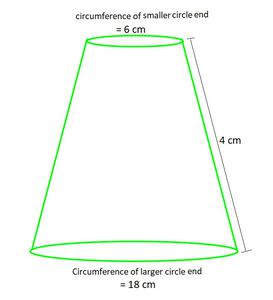Circumference of circle = 2π × (radius of circle)

Circumference of larger circle = 2πR

18 cm2 = 2πR

R =R =cm

Circumference of smaller circle = 2πr

6 cm2 = 2πr

r =r =cm

Now, as curve surface area of frustum = π (r+R) l

= π × () × 4

= 12 × 4                         (Taking π common and canceling it)

= 48cm2

Hence, the curved surface area of the frustum = 48cm2

### Question 3. A fez, the cap used by the Turks, is shaped like the frustum of a cone (see Fig.). If its radius on the open side is 10 cm, radius at the upper base is 4 cm and its slant height is 15 cm, find the area of material used for making it.

Solution:

Given values:

Slant height of frustum (l)= 15 cm

Let radius of smaller circle end (r) = 4 cm

Let radius of larger circle end (R) = 10 cm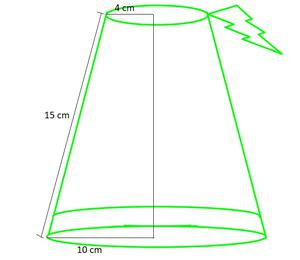Area of material used for making it = Curve surface area + area of upper base

= (π(r+R)l) + (πr2)

= π ((r+R)l + r2)                                              (Taking π common)

= π ((4+10) × 15 + (4 × 4))× (226)                                         (Taking π =)

= 710.286 cm2

Hence, the area of material used for making it = 710.286 cm

### Question 4. A container, opened from the top and made up of a metal sheet, is in the form of a frustum of a cone of height 16 cm with radii of its lower and upper ends as 8 cm and 20 cm, respectively. Find the cost of the milk which can completely fill the container, at the rate of ₹ 20 per litre. Also find the cost of metal sheet used to make the container, if it costs ₹ 8 per 100 cm2. (Take π = 3.14)

Solution:

Given values:

Height of frustum (h)= 16 cm

Let radius of smaller circle end (r) = 8 cm

Let radius of larger circle end (R) = 20 cm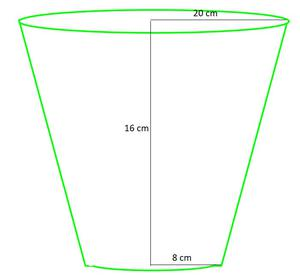The amount of milk to fill the container = Volume of frustumπh (r2 + R2 + rR)× 3.14 × 16 (8×8 + 20×20 + 8×20)                (Taking π=3.14)× 3.14 × 16 × (624)

= 10449.92 cm3

Cost of 1 litre milk = ₹ 20

And as, 1 m3 = 1000 cm3 = 1 litre

10449.92 cm3 = () ×10449.92 litres

cost of 10449.92 cm3 = () × 20

= ₹ 208.998

Now, metal sheet used to make the container = Curve surface area + area of lower base

= (π(r+R)l) + (πr2)

= π ((r+R)l + r2)                                              (Taking π common)

= π ((20+8) × (√(162+(20-8)2)) + (8 × 8))                                (Slant height (l) = √(h2+(R-r)2))

= 3.14 × (28 × √400 + 64)                                       (Taking π = 3.14)

= 3.14 × (624)

= 1959.36 cm2

Hence, the metal sheet used to make the container = 1959.36 cm2

As, cost of 100 cm2= ₹ 8

1959.36 cm2 = (8/100) × 1959.36

= ₹156.748

Hence, the cost of the milk which can completely fill the container = ₹ 208.998

and, the cost of metal sheet used to make the container = ₹156.748

### Question 5. A metallic right circular cone 20 cm high and whose vertical angle is 60° is cut into two parts at the middle of its height by a plane parallel to its base. If the frustum so obtained be drawn into a wire of diameter 1/16 cm, find the length of the wire.

Solution:

As the angle is cut into two equal parts, the height gets half too.

Let radius of smaller circle end = r

Let radius of larger circle end = R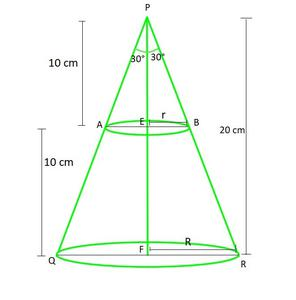In ∆PFR and ∆PEB

tan ∝ =tan 30° =R =r =and as height of frustum = 10 cm

So according to the question,

Frustum is converted to cylindrical wire having diametercm

Volume of Frustum = Volume of Cylinder

Volume of Frustum =πh (r2 + R2 + rR)cm3  ………………………..(1)

Volume of Cylinder =π(radius)2Hπ()2H …………………(2)

As (1) = (2) , then

7000π / 9 = 1/3 π(1/(16×2))2H

H =(cancel π from both side)

H = 796444.443 cm

H = 7964.44 m

Hence, the length of the wire = 7964.44 m